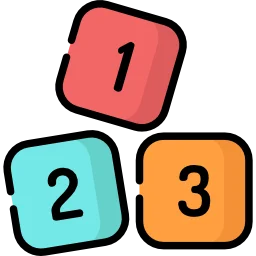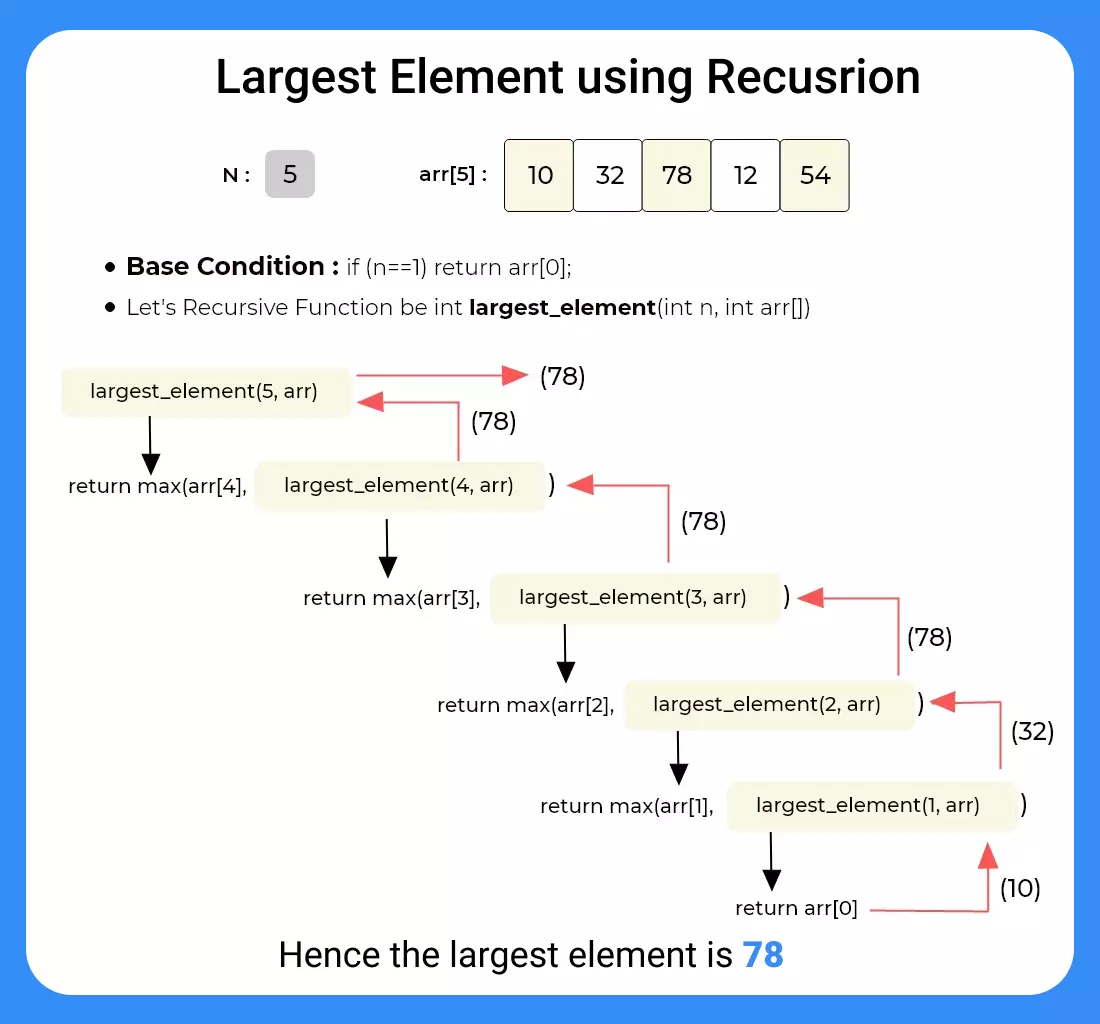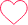# Recursive Program to find Largest Element of the array in Java

## Largest Element of  the array using Recursion in Java

Here, in this page we will discuss the program to find the largest element of the array using recursion in Java programming language. We are given with an array and we need to print the largest element among the elements of the array.

Example :

• Input : arr = {13, 89, 76, 43, 7, 90}
• Output : Largest Element is 90

We will discuss both approaches to find largest element using recursion and iteratively.## Method 1(Using Recursion) :

• Create a recursive function say, largest_element (int n, int arr[]).
• Base Condition : If(n==1) return arr. ( If the remaining array is of length 1, return the only present element i.e. arr )
• Else, return max(arr[n-1], largest_element(n-1, arr))
• If the base case is not met, then call the function by passing the array of one size less from the end, i.e. from arr to arr[n-1].## Code in Java (largest element of the array using recursion in Java)

Run
```//Largest Element of the array using Recursion in Java
public class Main {
static int arr[] = {10, 324, 45, 90, 98};
// Method to find maximum element in arr[]
static int largest() {
int i;
// Initialize maximum element
int max = arr;
// Traverse array elements from second and compare every element with current max
for (i = 1; i < arr.length; i++)
if (arr[i] > max) max = arr[i];
return max;
}
public static void main(String[] args) {
System.out.println("Largest in given array is " + largest());
}
}```

#### Output

`Largest in given array is 324`

## Method 2: (Non Recursive Approach)

• Create a variable say max_element and initialize with INT_MIN.
• Run a loop from 0 to N and set max_element = max(arr[i], max_element).
• After complete iteration print max_element.
Run
```//Largest Element of the array using Recursion in Java
public class Main
{
static int arr[] = {10, 32, 45, 90, 98};
// Method to find maximum in arr[]
static int largest()
{
int i;
// Initialize maximum element
int max = arr;

// Traverse array elements from second and compare every element with current max
for (i = 1; i < arr.length; i++)                        if (arr[i] > max)
max = arr[i];
return max;
}
public static void main(String[] args)
{
System.out.println("Largest in given array is " + largest());
}
}```
`Largest in given array is 98`

### Related Banners

Get PrepInsta Prime & get Access to all 200+ courses offered by PrepInsta in One Subscription

### 2 comments on “Recursive Program to find Largest Element of the array in Java”

•Mayank Tambe

//recrusive way in simpler way
public class Main
{
public static void main(String[] args) {

recurse obj=new recurse();
int arr[]={100,4,200,9,10};
System.out.println(obj.largest(arr,4));

}
}
class recurse{
int big=0;
public int largest(int arr[],int n){

if(nbig)
big=arr[n];

return largest(arr,n – 1);

}
}0
•Ravindra

public static int maxElement(int[] arr, int i, int max){
if (i==arr.length){
return max;
}
if (max<arr[i]){
max = arr[i];
}
return maxElement(arr,i+1,max);
}0